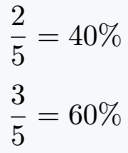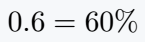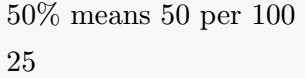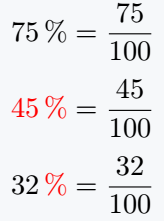# How to write percent(%) symbol in LaTeX?

If you use backslash \ before the percent % symbol in latex, you will return % symbol as the output.

And this is the easiest way to represent % symbol in a document.

Symbol Percent
Type of symbol Operator
Package No
Argument No
Latex command \%
Example A \% B → A % B
\documentclass{article}
\begin{document}
$\frac{2}{5} = 40\%$
$\frac{3}{5} = 60\%$
\end{document}


Output :By mistake, you will always try to use the keyboard’s percent symbol indirect text. However, as a result, you will not see any percentage symbol as output.

\documentclass{article}
\begin{document}
% latex percent symbol
$0.6 = 60\%$
\end{document}


Output :Because % symbol in latex is only used to represent single line comment.

## Insert percentage symbol in text

In the case of text mode, you need to add  \ symbol before % symbol. There is no separate rule for this.

If you use only a percentage(%) symbol in a text, the text on the right side of the percentage symbol will be converted to a single-line comment.

\documentclass{article}
\begin{document}
50\% means 50 per 100 \$4pt] 25% means 25 per 100 \end{document}  Output :## Use siunitx package for value and percentage symbol In this package, the percent symbol has been considered as SI Unit. As a result, you can print the value and symbol together in the document. \documentclass{article} \usepackage{siunitx} \begin{document} \[ \qty{75}{\percent} = \frac{75}{100}$
$\qty[color=red]{45}{\percent} = \frac{45}{100}$
$\qty[unit-color=red]{32}{\percent} = \frac{32}{100}$
\end{document}


Output :#### Md Jidan Mondal

LaTeX expert with over 10 years of experience in document preparation and typesetting. Specializes in creating professional documents, reports, and presentations using LaTeX.